Divisor class group

The quotient group of the groupof divisorial ideals (cf. Divisorial ideal) of a Krull ringby the subgroup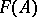consisting of the principal ideals. The divisor class group is Abelian and is usually denoted by. The group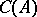is generated by the classes of the prime ideals of height 1 in(cf. Height of an ideal).

In a sense, the divisor class group measures the divergence from uniqueness of the factorization of elements ofinto irreducible factors. Thus, a factorial ring has trivial divisor class group.

Letbe a homomorphism of Krull rings; then, under certain additional assumptions (for instance, whenis an integral or flat extension of), there is a canonical homomorphism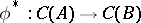of divisor class groups. Ifis the localization ofwith respect to a multiplicative system(cf. Localization in a commutative algebra), then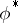is surjective and the kernel ofis generated by the divisorial prime ideals ofthat meet(Nagata's theorem). Ifis the ring of polynomials over, then the canonical homomorphismis bijective (this is a generalization of Gauss' theorem stating that the ring of polynomials over a field is factorial). In the more general case whereis the symmetric Noetherian algebra of an-module, the canonical homomorphismis bijective provided that all symmetric powersare reflexive. Ifis the ring of formal power series over, thenis injective (and even left invertible), but not bijective, in general.

The subgroup ofgenerated by the invertible ideals is isomorphic to the Picard group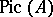of, and the functorial properties ofandare compatible. Thus, ifis a faithfully flat extension of a ringand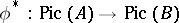is injective, thenis also injective. In particular, if the completion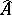of a local ringis factorial, thenis also factorial (Mori's theorem).

Letbe a normal Noetherian ring. The groupcoincides withif and only ifis locally a factorial ring, that is, if all the local rings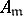are factorial (for instance, whenis regular). More exactly, if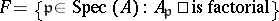, then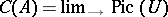, whereruns over the system of open subschemes of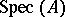containing. This allows one to define the divisor class group of a normal scheme  — the Weil divisor class group (see Divisor).

Divisor class groups were first studied for rings of algebraic numbers, and the earliest results concerning the finiteness of these groups were obtained by E. Kummer. There is a close connection between the properties of the divisor class group and number-theoretical problems, for instance, Fermat's theorem. Tables of orders of divisor class groups of certain rings of algebraic numbers are provided in .

In full generality, the theory of divisor class groups was obtained by W. Krull; P. Samuel studied the functorial character of divisor class groups and proposed some methods for computing them (for example, the method of descent). Other approaches to the study of the divisor class group are based on comparison with the Picard group, and cohomological and algebraic-geometrical methods are applied as well.

Every Abelian group occurs as a divisor class group.